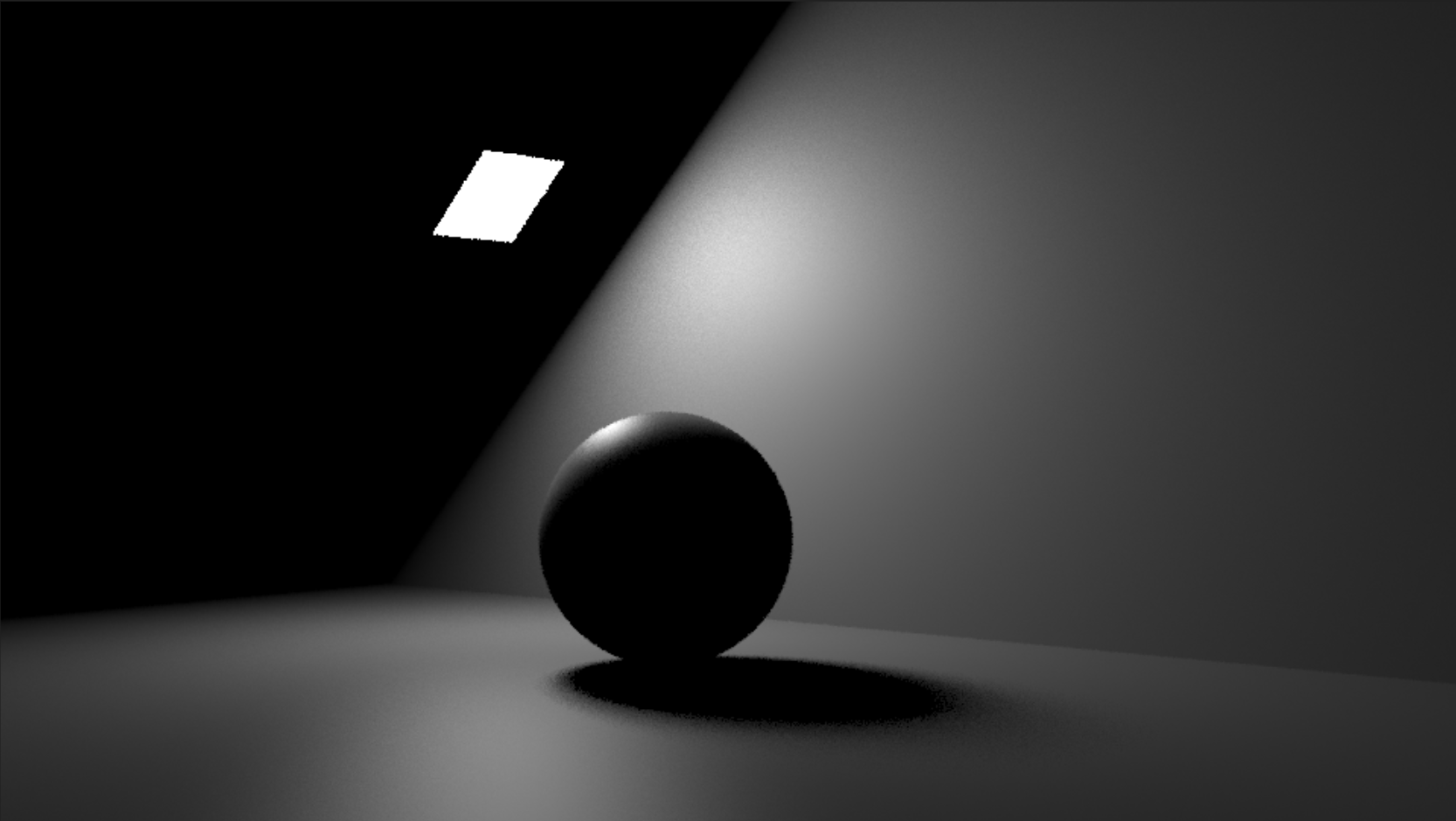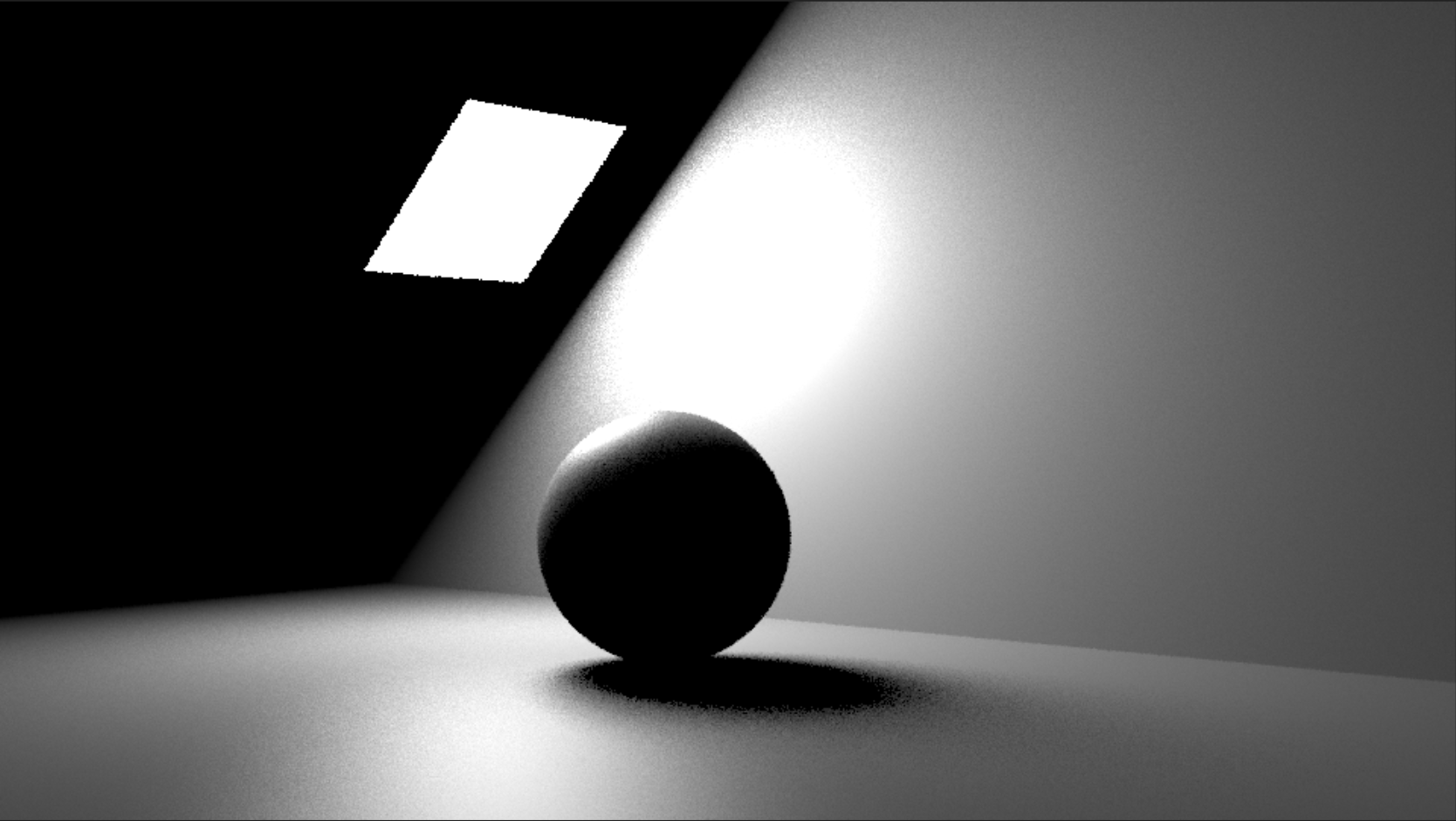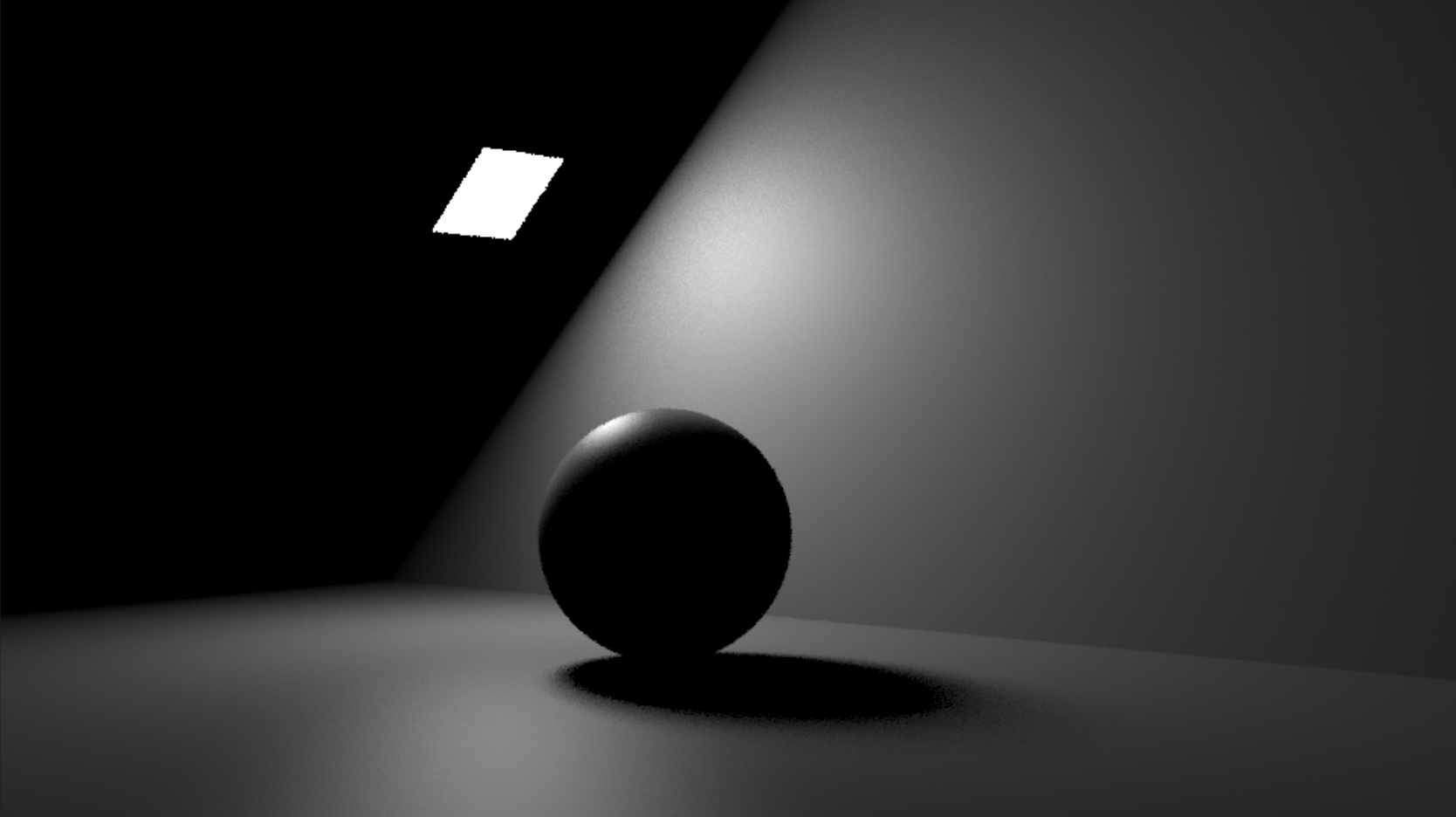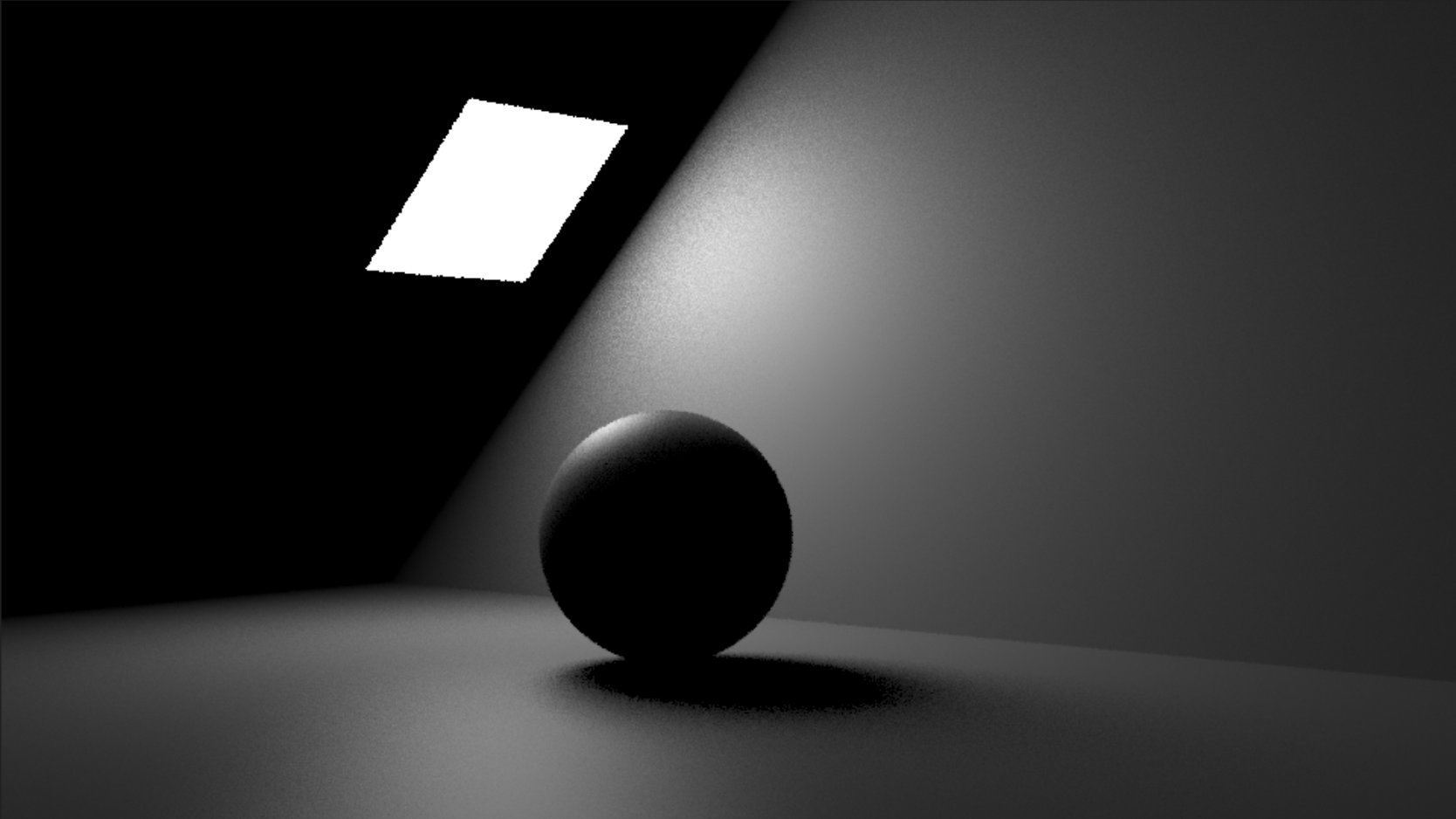# Light Normalization

By default, all lights in MoonRay are `normalized`, which means that a light’s brightness is not affected by its size.

## When normalized is false …

… we calculate the radiance as follows:

``````// not normalized
radiance = color * intensity * 2^(exposure)
``````

As the light’s surface area increases, the amount of radiance it produces increases as well. In the example below, the light on the right is 4x the size of the light on the left. As such, with normalized off, the light on the right is 4x brighter than the light on the left.normalized = false
width = 1
height = 1
intensity = 20
normalized = false
width = 2
height = 2
intensity = 20

## When normalized is true…

… we divide the radiance by the surface area to ensure that the amount of light energy is not affected by the size of the light.

``````// normalized
radiance = color * intensity * 2^(exposure) / surface_area
``````normalized = true
width = 1
height = 1
intensity = 20
normalized = true
width = 2
height = 2
intensity = 20

Note that the amount of light given off by the larger area light on the right is the same as that given off by the unit area light on the left. Basically, the radiance given off by the larger light has been divided by 4. We can confirm this by comparing our larger area light un-normalized with intensity 5 to the same area light normalized with intensity 20.normalized = false
width = 2
height = 2
intensity = 5
normalized = true
width = 2
height = 2
intensity = 20

## Should normalized be true or false?

• For most area lights, it should probably be true, because you likely don’t want light to become brighter as it grows
• For MeshLights, it should probably be false, as you do want the light to become brighter as it grows or adds more disconnected parts
• If you want MeshLights to behave the same as emissive geometry, set normalized to false